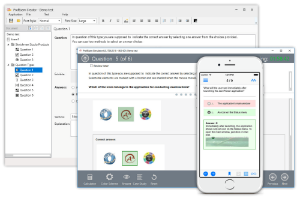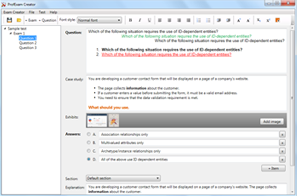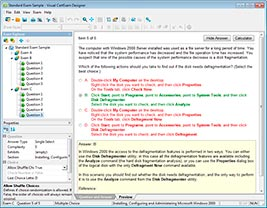# Download Java SE 8 Programmer II.dump4pass.1Z0-809.2021-06-06.1e.207q.vcex

## File Info

 Exam Java SE 8 Programmer II Number 1z0-809 File Name Java SE 8 Programmer II.dump4pass.1Z0-809.2021-06-06.1e.207q.vcex Size 8.46 Mb Posted June 06, 2021 Downloads 5

### How to open VCEX & EXAM Files?

Files with VCEX & EXAM extensions can be opened by ProfExam Simulator.

Coupon: EXAMFILESCOM
With discount: 20%

## Demo Questions

Question 1
Given the definition of the Vehicle class:
Class Vehhicle {
int distance;//line n1
Vehicle (int x) {
this distance = x;
}
public void increSpeed(int time)   {    //line n2
int timeTravel = time;            //line n3
class Car {
int value = 0;
public void speed () {
value = distance /timeTravel;
System.out.println (“Velocity with new speed”+value+”kmph”);

new Car().speed();
}

and this code fragment:
Vehicle v = new Vehicle (100);
v.increSpeed(60);
What is the result?

• A: Velocity with new speed
• B: A compilation error occurs at line n1.
• C: A compilation error occurs at line n2.
• D: A compilation error occurs at line n3.

Question 2
Given:
IntStream stream = IntStream.of (1,2,3);
IntFunction<Integer> inFu= x -> y -> x*y;//line n1
IntStream newStream = stream.map(inFu.apply(10));    //line n2
newStream.forEach(System.output::print);
Which modification enables the code fragment to compile?

• A: Replace line n1 with:
IntFunction<UnaryOperator> inFu = x -> y -> x*y;
• B: Replace line n1 with:
IntFunction<IntUnaryOperator> inFu = x -> y -> x*y;
• C: Replace line n1 with:
BiFunction<IntUnaryOperator> inFu = x -> y -> x*y;
• D: Replace line n2 with:
IntStream newStream = stream.map(inFu.applyAsInt (10));

Question 3
Given the code fragment:
List<Integer> values = Arrays.asList (1, 2, 3);
values.stream ()
.map(n -> n*2)        //line n1
.peek(System.out::print)    //line n2
.count();
What is the result?

• A: 246
• B: The code produces no output.
• C: A compilation error occurs at line n1.
• D: A compilation error occurs at line n2.

Question 4
Given the code fragment:
public class Foo {
public static void main (String [ ] args) {
Map<Integer, String> unsortMap = new HashMap< > ( );
unsortMap.put (10, “z”);
unsortMap.put (5, “b”);
unsortMap.put (1, “d”);
unsortMap.put (7, “e”);
unsortMap.put (50, “j”);
Map<Integer, String> treeMap = new TreeMap <Integer, String> (new
Comparator<Integer> ( )  {
@Override public int compare (Integer o1, Integer o2) {return
o2.compareTo
(o1); }  } );
treeMap.putAll (unsortMap);
for (Map.Entry<Integer, String> entry : treeMap.entrySet () ) {
System.out.print (entry.getValue () + “ “);

}

What is the result?

• A: A compilation error occurs.
• B: d b e z j
• C: j z e b d
• D: z b d e j

Question 5
Which two reasons should you use interfaces instead of abstract classes? (Choose two.)

• A: You expect that classes that implement your interfaces have many common methods or fields, or require access modifiers other than public.
• B: You expect that unrelated classes would implement your interfaces.
• C: You want to share code among several closely related classes.
• D: You want to declare non-static on non-final fields.
• E: You want to take advantage of multiple inheritance of type.

Question 6
Given:
public class Counter  {
public static void main (String[ ] args) {
int a = 10;
int b = -1;
assert (b >=1) : “Invalid Denominator”;
int ɫ = a / b;
System.out.println (c);
}

What is the result of running the code with the –ea option?

• A: -10
• B: 0
• C: An AssertionError is thrown.
• D: A compilation error occurs.

Question 7
Given:
class Bird  {
public void fly ()  {  System.out.print(“Can fly”); }

class Penguin extends Bird  {
public void fly ()  {  System.out.print(“Cannot fly”); }

and the code fragment:
class Birdie {
public static void main (String [ ] args)  {
fly( ( ) -> new Bird ( ));
fly (Penguin : : new);
}
/* line n1 */

Which code fragment, when inserted at line n1, enables the Birdie class to compile?

• A: static void fly (Consumer<Bird> bird) {
bird :: fly ();
}
• B: static void fly (Consumer<? extends Bird> bird) {
bird.accept( ) fly ();
}
• C: static void fly (Supplier<Bird> bird) {
bird.get( ) fly ();
}
• D: static void fly (Supplier<? extends Bird> bird) {
LOST

Question 8
Given:
1. abstract class Shape  {
2.     Shape ( )   { System.out.println  (“Shape”);     }
3.     protected void area ( ) { System.out.println  (“Shape”);     }
4. }
5.
6. class Square extends Shape  {
7.     int side;
8.     Square int side {
9.        /* insert code here */
10.         this.side = side;
11.     }
12.     public void area ( ) { System.out.println  (“Square”);     }
13.     }
14. class Rectangle extends Square {
15.     int len, br;
16.     Rectangle (int x, int y)   {
17.         /* insert code here */
18.         len = x, br = y;
19.     }
20. void area ( ) { System.out.println  (“Rectangle”);     }
21. }
Which two modifications enable the code to compile? (Choose two.)

• A: At line 1, remove abstract
• B: At line 9, insert super ( );
• C: At line 12, remove public
• D: At line 17, insert super (x);
• E: At line 17, insert super (); super.side = x;
• F: At line 20, use public void area ( ) {

Question 9
Given:
class Sum extends RecursiveAction   {                 //line n1
static final int THRESHOLD_SIZE  = 3;
int stIndex, lstIndex;
int [ ] data;
public Sum (int [ ]data, int start, int end)   {
this.data = data;
this stIndex = start;
this. lstIndex = end;
}
protected void compute ( )    {
int sum = 0;
if (lstIndex – stIndex <= THRESHOLD_SIZE)  {
for (int i = stIndex; i < lstIndex; i++)   {
sum += data [i];
}
System.out.println(sum);
} else {
new Sum (data, stIndex + THRESHOLD_SIZE, lstIndex).fork( );
new Sum (data, stIndex,
Math.min  (lstIndex, stIndex + THRESHOLD_SIZE)
).compute ();
}
}

and the code fragment:
ForkJoinPool fjPool = new ForkJoinPool ( );
int data [ ] = {1, 2, 3, 4, 5, 6, 7, 8, 9, 10}
fjPool.invoke (new Sum (data, 0, data.length));
and given that the sum of all integers from 1 to 10 is 55.
Which statement is true?

• A: The program prints several values that total 55.
• B: The program prints 55.
• C: A compilation error occurs at line n1.
• D: The program prints several values whose sum exceeds 55.

Question 10
Given the content of Operator.java, EngineOperator.java, and Engine.java files:and the code fragment:What is the result?

• A: The Engine.java file fails to compile.
• B: The EngineOperator.java file fails to compile.
• C: The Operator.java file fails to compile.
• D: ON OFF

CONNECT US##### PROFEXAM WITH A 20% DISCOUNT

You can buy ProfExam with a 20% discount..

HOW TO OPEN VCEX AND EXAM FILES

Use ProfExam Simulator to open VCEX and EXAM filesHOW TO OPEN VCE FILES

Use VCE Exam Simulator to open VCE files